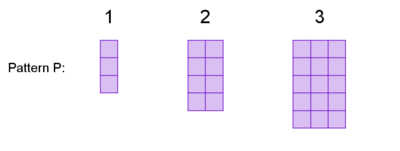##### Engage your students with effective distance learning resources. ACCESS RESOURCES>>

Alignments to Content Standards: A-REI.B.4.b

The first three steps of twoÂ visual patterns are shown below.The number of tiles in step $n$ of Pattern PÂ is defined by $p(n)=n^2+2n$. The number of tiles in step $n$ of Pattern QÂ is defined by $q(n)=2(n^2+4n)+14$.Â

1. For each pattern, decide whether there is a step with 399Â tiles in it. If so, which step is it? If not, explain how you know.
2. For each pattern, decide whether there is a step with 134Â tiles in it. If so, which step is it? If not, explain how you know.

## IM Commentary

This task belongs to aÂ series of threeÂ tasks that has students process a sequence of tile figures with the property that the $n$-th figure in the sequence has $f(n)$ tiles, for some quadratic function $f$. Students are asked to analyze the functions in the context of questions about the sequence of figures, a process which involves manipulating the quadratic expressions into different forms (identifying square roots at first, then completing the square in the third task). With solving quadratics, there can beÂ an impulse to put everything in standard form and just use the quadratic formula. However, seeing structure is emphasized in the standards because of how it connects and helps inÂ understanding many foundational concepts, and these tasks develop the ability to see structure when working with quadratic expressions and equations.Â

That the domain of these quadraticÂ functions isÂ the set of positive integers provides an interesting wrinkle which students might not be used to thinking about in the setting of quadratic functions.Â The functions roughly increase in complexity through the threeÂ tasks, with the intent that the techniques learned in each will be used and expanded in the subsequent tasks.Â The other tasks in the sequence are Quadratic Sequence 1Â and Quadratic Sequence 2.

Ideally, students will have had some experience working with visual patterns prior to this task. If not, students might benefit from additional questions to familiarize themselves with the idea. For example, they might be asked to draw the next step in the pattern.Â Or students could be asked to discuss how they "see" the pattern growing (here is a good primerÂ onÂ what that might look like.) OrÂ they could be asked to evaluate p(1) and explain its meaning in terms of the number of tiles in Pattern P.

The purpose of this task is to motivate solving a quadratic equation where the variable of interest, $n$, can not be isolatedÂ directlyÂ by "undoing" operations. Instead, students must tackle solving these equations in some other way. In previous tasks in the sequence, students could "undo" a squaring operation. So a natural way forward might be to think about how they could rewrite the equation with one sideÂ a perfect square, and that is the direction shown in the solution. This task could be used to motivate learning a more general procedure for solving by completing the square.

This task was inspired by visualpatterns.org.

## Solution

1. To see if pattern P has a step with 399Â tiles, we need to try to solve $n^2+2n=399$. Unlike in previous tasks in this sequence, we can't isolate $n$ by undoing operations, so we need another approach. In previous tasks in the sequence, we could "undo" a perfectÂ square on one side of the equation. Here, we might notice that the left side would be a perfect square if we added a $1$ to both sides, creatingÂ $n^2+2n+1=400$, which can be rewritten $(n+1)^2=400$. Since the square of $n+1$ is $400$, it must be the case that $n+1=20$, so $n=19$. Since $p(19)=399$, we conclude that the 19th step of pattern P has 399 tiles.
2. Does pattern P have a step with 134 tiles? Let's check $n^2+2n=134.$ Using the same trick as before, we rewrite that as $n^2+2n+1=135$ and $(n+1)^2=135$. Since 135 is not a perfect square, this doesn't have integer solutions, and we conclude that pattern P does not have a step with 134 tiles.

To check pattern Q, we try to solve $2(n^2+4n)+14=134$. Let's try to make it a perfect square equal to some number. We'll proceed as follows:$$2(n^2+4n)+14=134$$ $$2(n^2+4n)=120$$ $$n^2+4n=60$$ We can turn the left side into a perfect square if we add $4$ to both sides. $$n^2+4n+4=64$$ $$(n+2)^2=64$$ If that is true, then $n+2=8$, and $n=6$. Since $q(6)=134$, the sixth step of pattern Q has 134 tiles.